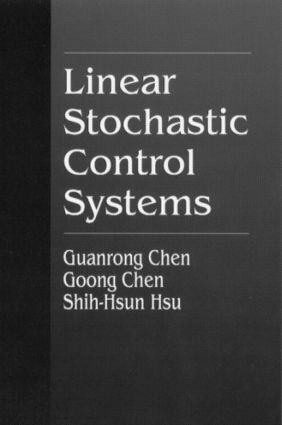Linear Stochastic Control Systems

1st Edition

CRC Press

400 pages

Purchasing Options:\$ = USD
Hardback: 9780849380754
pub: 1995-07-12
Currently out of stock
\$265.00
x

FREE Standard Shipping!

Description

Linear Stochastic Control Systems presents a thorough description of the mathematical theory and fundamental principles of linear stochastic control systems. Both continuous-time and discrete-time systems are thoroughly covered.

Reviews of the modern probability and random processes theories and the Itô stochastic differential equations are provided. Discrete-time stochastic systems theory, optimal estimation and Kalman filtering, and optimal stochastic control theory are studied in detail. A modern treatment of these same topics for continuous-time stochastic control systems is included. The text is written in an easy-to-understand style, and the reader needs only to have a background of elementary real analysis and linear deterministic systems theory to comprehend the subject matter.

This graduate textbook is also suitable for self-study, professional training, and as a handy research reference. Linear Stochastic Control Systems is self-contained and provides a step-by-step development of the theory, with many illustrative examples, exercises, and engineering applications.

Preface

Introduction

From Deterministic to Stochastic Linear Control Systems

Text Organization and Reading Suggestion

MATHEMATICAL PRELIMINARIES

Probability and Random Processes

Probability, Measure, and Integration

Convergence of Random Sequences

Random Vectors and Conditional Expectations

Second Order Processes and Calculus in Mean Square

Exercises

References

Itô Integrals and Stochastic Differential Equations

Markov Processes

Orthogonal Increments Processes and the Wiener-Lévy Process

Itô Integrals and Stochastic Differential Equations

Exercises

References

LINEAR STOCHASTIC CONTROL SYSTEMS: THE DISCRETE-TIME CASE

Analysis of Discrete-Time Linear Stochastic Control Systems

Analysis of Discrete-Time Causal LTI Systems

Analysis of Causal LTI Stochastic Control Systems

Analysis of the "State" Description of Controlled Markov Chains

State Space Systems and ARMA Models

Mathematical Modeling and Applications

Exercises

References

Optimal Estimation for Discrete-Time Linear Stochastic Systems

Optimal State Estimation

Recursive Optimal Estimation and Kalman Filtering

Modified Kalman Filtering Algorithms

Exercises

References

Optimal Control of Discrete-Time Linear Stochastic Systems

Introduction

Dynamic Programming and LQC Control Problems

LQC Optimal Control Problems

Exercises

References

LINEAR STOCHASTIC CONTROL SYSTEMS: THE CONTINUOUS-TIME CASE

Continuous-Time Linear Stochastic Control Systems

Analysis of Continuous-Time Causal LTI Systems

Further Discussion of Markov Processes

Dynamic Programming and LQ Control Problems

Exercises

References

Optimal Control of Continuous-Time Linear Stochastic Systems

The Continuous-Time LQ Stochastic Control Problem

Stochastic Dynamic Programming

Innovation Processes and the Kalman-Bucy Filter

Optimal Prediction and Smoothing

The Separation Principle

Exercises

References

Stability Analysis of Stochastic Differential Equations

Stability of Deterministic Systems

Stability of Stochastic Systems

Stability of Moments

Exercises

References

Appendix

Fundamental Real and Functional Analysis

Fundamental Matrix Theory and Vector Calculations

Martingales

References

Index